# 1. What should I do?

In this project, I need to implement the extended Kalman filter in C++, then using this filter to detect a bicycle that travels around the car. This is the image that detected by the EKF,Lidar measurements are red circles, radar measurements are blue circles with an arrow pointing in the direction of the observed angle, and estimation markers are green triangles.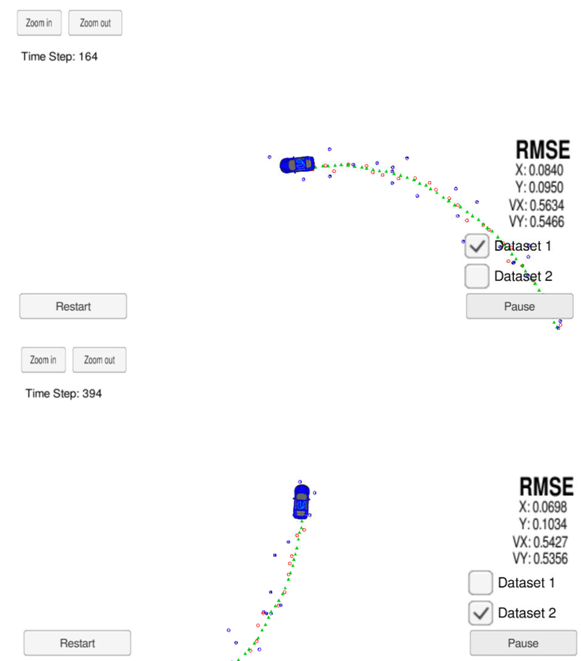# 2. Project sturcture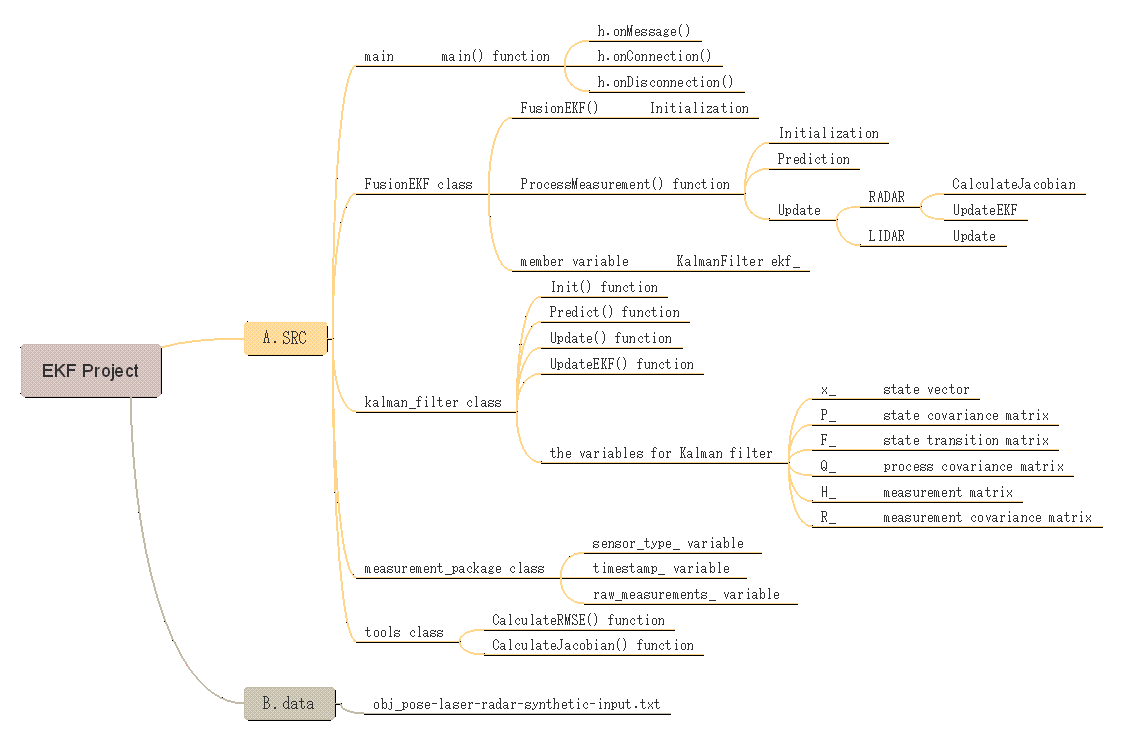# 3. For more details about the code

Summary of What Needs to Be Done:

• tools.cpp:RMSE and the Jacobian matrix.
• FusionEKF.cpp:initialize the Kalman Filter,prepare the Q and F matrices , and call the radar and lidar predict/update functions.
• kalman_filter.cpp:fill out the Predict(), Update(), and UpdateEKF() functions.

## 3.1 tools::RMSE()

Check the filter’s performance in terms of Root Mean Squared Error equation.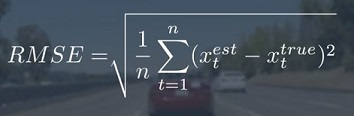``````VectorXd Tools::CalculateRMSE(const vector<VectorXd> &estimations,
const vector<VectorXd> &ground_truth) {
/**
* TODO: Calculate the RMSE here.
*/
VectorXd rmse(4);
rmse << 0,0,0,0;

// check the validity of the following inputs:
//  * the estimation vector size should not be zero
//  * the estimation vector size should equal ground truth vector size
if (estimations.size() != ground_truth.size()
|| estimations.size() == 0) {
cout << "Invalid estimation or ground_truth data" << endl;
return rmse;
}

// accumulate squared residuals
for (unsigned int i=0; i < estimations.size(); ++i) {
VectorXd residual = estimations[i] - ground_truth[i];
// coefficient-wise multiplication
residual = residual.array()*residual.array();
rmse += residual;
}

// calculate the mean
rmse = rmse/estimations.size();

// calculate the squared root
rmse = rmse.array().sqrt();

// return the result
return rmse;
}
``````

## 3.2 tools::CalculateJacobian()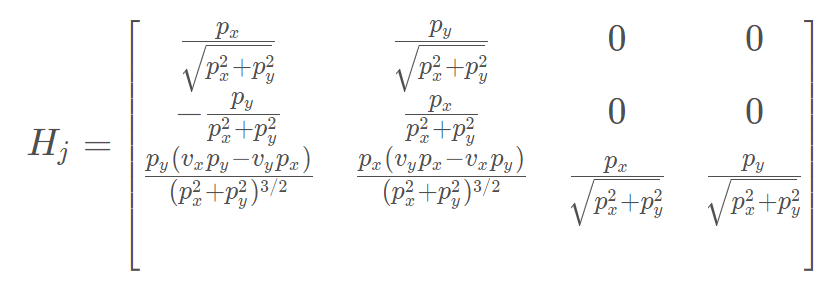Calculate the Jacobian matrix Hj using the above equation.

``````MatrixXd Tools::CalculateJacobian(const VectorXd& x_state) {
/**
* TODO:
* Calculate a Jacobian here.
*/
MatrixXd Hj(3,4);
// recover state parameters
float px = x_state(0);
float py = x_state(1);
float vx = x_state(2);
float vy = x_state(3);

// pre-compute a set of terms to avoid repeated calculation
float c1 = px*px+py*py;
float c2 = sqrt(c1);
float c3 = (c1*c2);

// check division by zero
if (fabs(c1) < 0.0001) {
cout << "CalculateJacobian () - Error - Division by Zero" << endl;
return Hj;
}

// compute the Jacobian matrix
Hj << (px/c2), (py/c2), 0, 0,
-(py/c1), (px/c1), 0, 0,
py*(vx*py - vy*px)/c3, px*(px*vy - py*vx)/c3, px/c2, py/c2;

return Hj;
}
``````

## 3.3 FusionEKF::ProcessMeasurement()

• Firstly, I need to initialize variables and matrices and the Kalman filter position vector with the first sensor measurements.

If this is the first measurement, the Kalman filter will try to initialize the object’s location with the sensor measurement.

``````  /**
* Initialization
*/
if (!is_initialized_) {
/**
* TODO: Initialize the state ekf_.x_ with the first measurement.
* TODO: Create the covariance matrix.
* You'll need to convert radar from polar to cartesian coordinates.
*/

// first measurement
cout << "EKF: " << endl;
ekf_.x_ = VectorXd(4);
ekf_.x_ << 1, 1, 1, 1;

//set the first measurement value
float first_measurement_x = measurement_pack.raw_measurements_;
float first_measurement_y = measurement_pack.raw_measurements_;

// TODO: Convert radar from polar to cartesian coordinates
//         and initialize state.
ekf_.x_ = first_measurement_x*cos(first_measurement_y);
ekf_.x_ = first_measurement_x*sin(first_measurement_y);
}
else if (measurement_pack.sensor_type_ == MeasurementPackage::LASER) {
// TODO: Initialize state.
ekf_.x_ = first_measurement_x;
ekf_.x_ = first_measurement_y;
}

//
ekf_.P_ = MatrixXd(4, 4);
ekf_.P_ << 10, 0, 0, 0,
0, 10, 0, 0,
0, 0, 1000, 0,
0, 0, 0, 1000;

previous_timestamp_ = measurement_pack.timestamp_;

//
ekf_.F_ = MatrixXd(4, 4);
ekf_.F_ << 1, 0, 1, 0,
0, 1, 0, 1,
0, 0, 1, 0,
0, 0, 0, 1;

// done initializing, no need to predict or update
is_initialized_ = true;
return;
}
``````
• secondly, modify the F and Q matrices prior to the prediction step based on the elapsed time between measurements。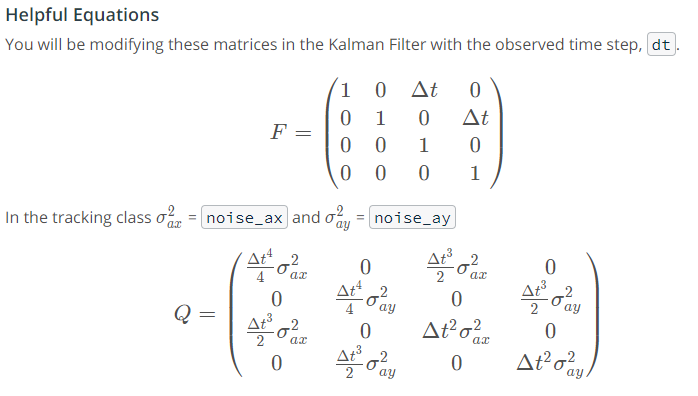``````  /**
* Prediction
*/

/**
* TODO: Update the state transition matrix F according to the new elapsed time.
* Time is measured in seconds.
* TODO: Update the process noise covariance matrix.
* Use noise_ax = 9 and noise_ay = 9 for your Q matrix.
*/
/*Taking into account the timestamp*/
float deltaT = measurement_pack.timestamp_ - previous_timestamp_;
//Converting time to seconds.
deltaT = deltaT/pow(10.0, 6);

//Setting previous timestamp to current timestamp
previous_timestamp_ = measurement_pack.timestamp_;

/*Initializing Process covariance matrix*/
float noise_ax = 9;
float noise_ay = 9;
float time_r2 = pow(deltaT, 2);
float time_r3 = pow(deltaT, 3);
float time_r4 = pow(deltaT, 4);

//Update F matrix to take into account deltaT for latest measurement received.
ekf_.F_.row(0) = deltaT;
ekf_.F_.row(1) = deltaT;

//Declare and fill state covariance matrix representing stocastic part of motion.
ekf_.Q_ = MatrixXd(4, 4);
ekf_.Q_ << time_r4*noise_ax/4, 0, time_r3*noise_ax/2, 0,
0, time_r4*noise_ay/4, 0, time_r3*noise_ay/2,
time_r3*noise_ax/2, 0, time_r2*noise_ax, 0,
0, time_r3*noise_ay/2, 0, time_r2*noise_ay;

ekf_.Predict();
``````
• At last, call the update step for either the lidar or radar sensor measurement. Because the update step for lidar and radar are slightly different, there are different functions for updating lidar and radar。
``````/**
* Update
*/

/**
* TODO:
* - Use the sensor type to perform the update step.
* - Update the state and covariance matrices.
*/

Hj_ = tools.CalculateJacobian(ekf_.x_);
ekf_.H_ = Hj_;
ekf_.UpdateEKF(measurement_pack.raw_measurements_);

} else {
ekf_.R_ = R_laser_;
ekf_.H_ = H_laser_;
ekf_.Update(measurement_pack.raw_measurements_);
}

``````

## 3.4 kalman_filter::Predict()

``````void KalmanFilter::Predict() {
/**
* TODO: predict the state
*/
x_ = F_ * x_;
MatrixXd Ft = F_.transpose();
P_ = F_ * P_ * Ft + Q_;
}
``````

## 3.5 kalman_filter::Update()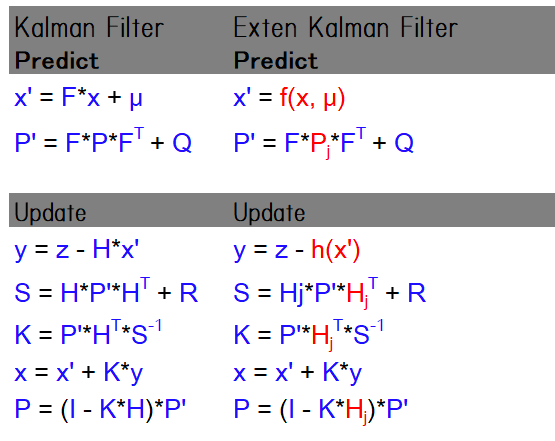``````void KalmanFilter::Update(const VectorXd &z) {
/**
* TODO: update the state by using Kalman Filter equations
*/
VectorXd z_pred = H_ * x_;
VectorXd y = z - z_pred;
MatrixXd Ht = H_.transpose();
MatrixXd S = H_ * P_ * Ht + R_;
MatrixXd Si = S.inverse();
MatrixXd PHt = P_ * Ht;
MatrixXd K = PHt * Si;

//new estimate
x_ = x_ + (K * y);
long x_size = x_.size();
MatrixXd I = MatrixXd::Identity(x_size, x_size);
P_ = (I - K * H_) * P_;
}
``````

## 3.6 kalman_filter::UpdateEKF()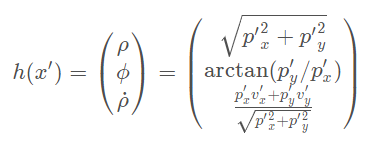``````void KalmanFilter::UpdateEKF(const VectorXd &z) {
/**
* TODO: update the state by using Extended Kalman Filter equations
*/
MatrixXd h = MatrixXd(z.rows(), z.cols());
//state parameters
float px = x_(0);
float py = x_(1);
float vx = x_(2);
float vy = x_(3);
float sqrt_px2_py2 = sqrt(pow(px, 2) + pow(py, 2));
//Prevent division by zero
if (sqrt_px2_py2 < 0.0001) {
sqrt_px2_py2 = 0.0001;
}

//Set measurement function
h << sqrt_px2_py2,
atan2(py, px),
(px * vx + py * vy)/sqrt_px2_py2;
VectorXd y = z - h;

//Normalize phi angle and bring it in the range (-pi, pi)
while (y > M_PI) {
y -= 2.0 * M_PI;
}
while (y <-M_PI) {
y += 2.0 * M_PI;
}

MatrixXd Ht = H_.transpose();
MatrixXd S = H_ * P_ * Ht + R_;
MatrixXd Si = S.inverse();
MatrixXd K = P_ * Ht * Si;

//new estimate
x_ = x_ + (K * y);
int x_size = x_.size();
MatrixXd I = Eigen::MatrixXd::Identity(x_size, x_size);
P_ = (I - (K * H_)) * P_;
}
``````

# 4. Reflection:

In fact, most of the code has been compeleted by the last assignment. In order to understand the EKF more deeply, I should implement it from the zero.